# How to Create a Running Total in Excel (Cumulative Sum)Agnes Lo

## What is a running total?

A running total in Excel (also known as cumulative sum) refers to the partial sum of a data set. It is a summation of a sequence of numbers that is refreshed every time a new number is added to the sequence.

Running totals are very commonly used in Excel, especially when daily data is involved such as daily sales, daily bank balance, daily calorie intake or the scores of a sports game. It reveals the total number for each day or month, depending on the measurements.

## How to create a running total

There are many ways to create a running total, including using simple addition, the SUM function, and Pivot Tables.

Let’s look at how to create a basic running total by using addition to familiarize you with the logic behind it.

Use this free Excel file to practice along with the tutorial.

Below is a credit card statement with various expenses and credits in the list. A running total helps keep track of the credit limit available and personal expenditures.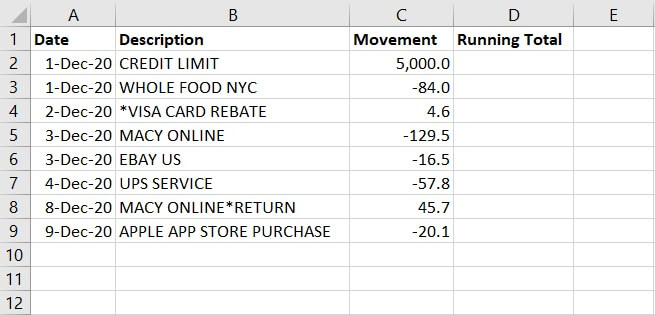To create a running total, click D2 and enter =C2, the beginning credit limit to start with.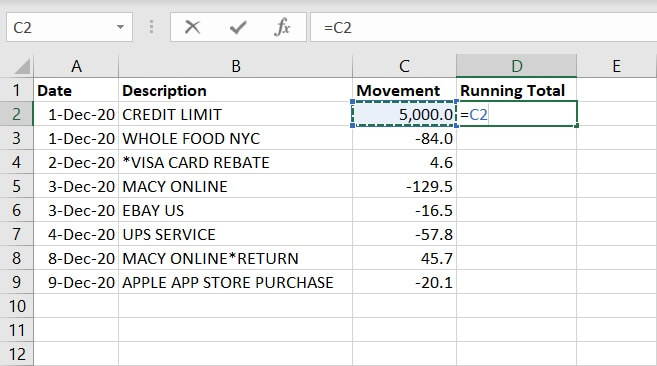Given that running totals reveal the summation of the data as new items are added to the total mix, to keep the changes:

• Click Cell D3, enter =D2+C3.

This is to add the beginning credit limit and the new item — an expense from Whole Foods.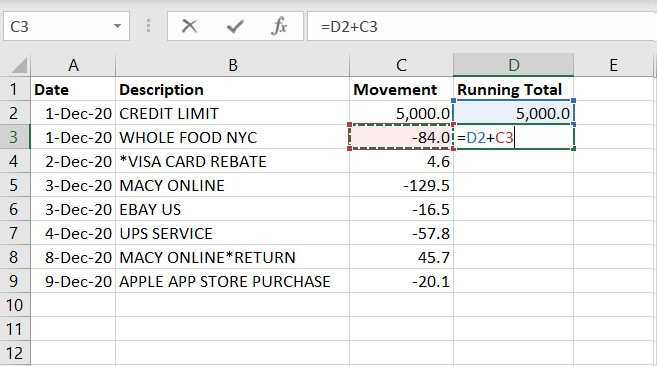Now Cell D3 indicates the credit limits after deducting the expense from Whole Foods — \$4916.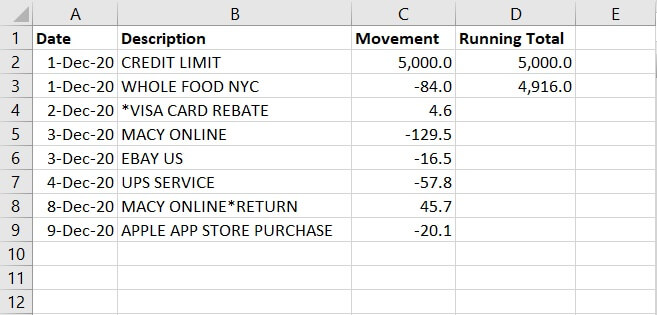To find out the remaining credit limits available, drag down the formula in D3 and apply it to the rest of the cells under column D.

From the formulas, you can tell that each value of the running total takes reference from the previous value of running total and adds on the value of the new item.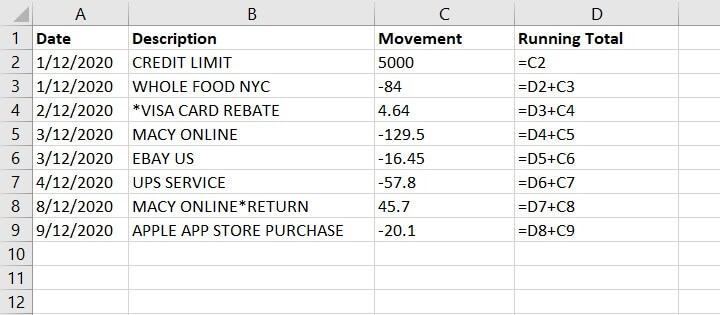Below is the full picture, with running total detailing the movement of the credit limit with each item added on.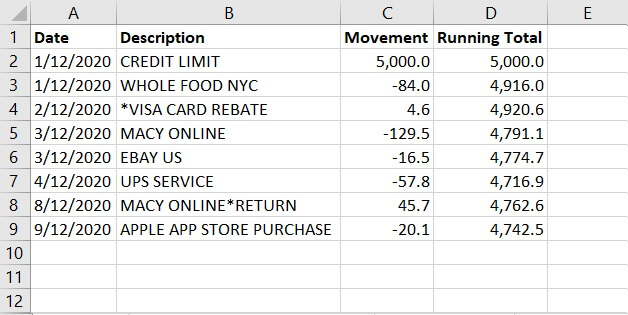## How to calculate a running total in Excel

As mentioned previously, there are multiple ways to calculate running totals in Excel, depending on the complexity of the situation on hand and the usage.

### 1. Create running total by using the SUM function

Creating a running total by using the SUM function is pretty similar to using simple addition.

In Cell D2, enter:

``=SUM(C2,D1)``

This is to add up the value of the beginning credit limit and the header — Running Total. The header contains no value itself, and will be considered as 0 in the calculation.

This, unlike addition, saves you from the extra work of creating the beginning balance first, then adding the new item in the second cell.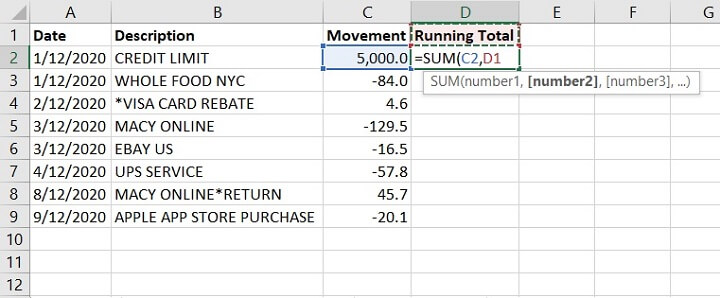Copy the formula in the rest of the cell. It should look like the image below, with each running total taking reference from the previous values.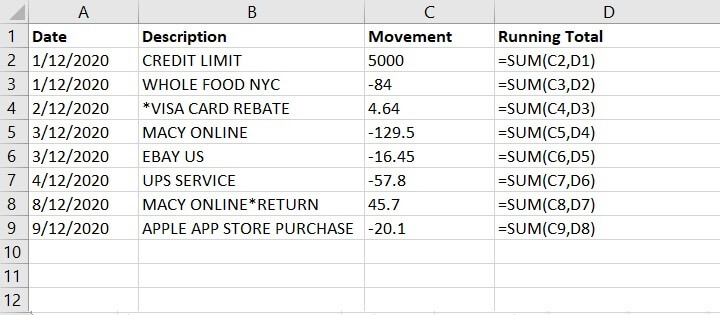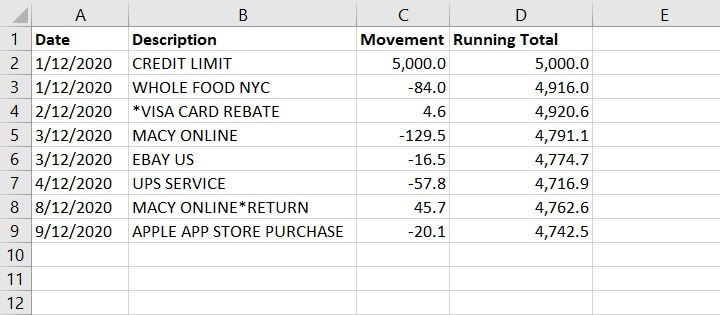Though, when a row is added, there will be a gap in the running total, and users will need to copy the formula and drag it down to refresh the rest.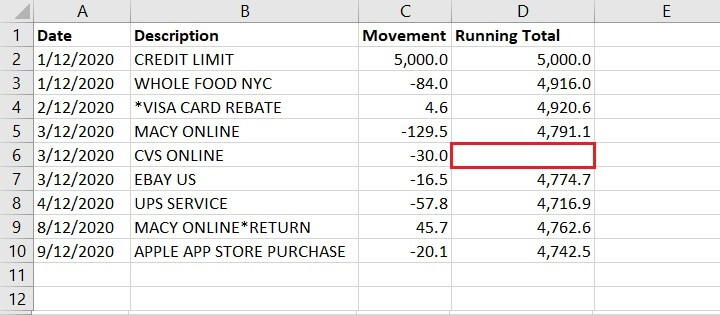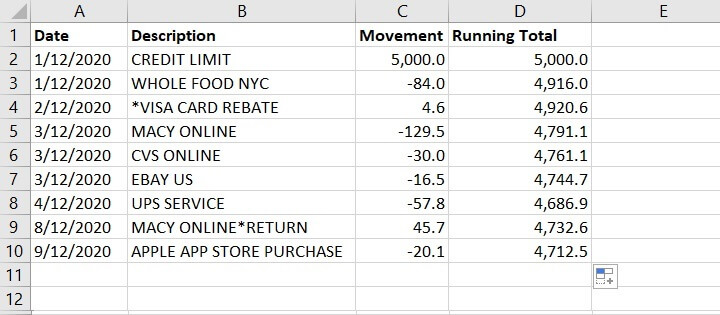Likewise, when a row is deleted, error #REF! shows as the cell reference is removed. To calculate the running total, copy the formula from the last correct cell (D5) and apply to the rest.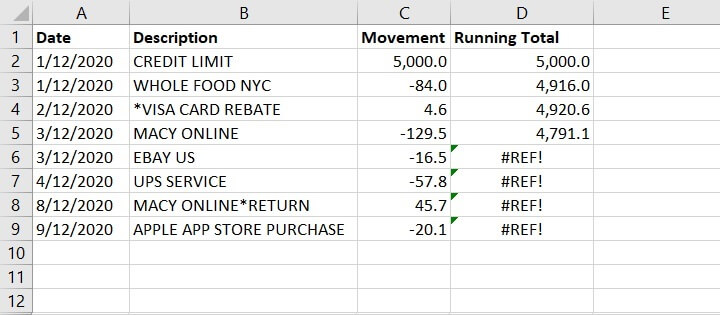The SUM function makes it quick and easy to calculate the running total. However, when adjustments to the data are required (e.g. adding or deleting a row) users will need to manually adjust and re-apply the correct formulas to the cells.

If the data set is small with a few calculations or sheets involved, manual adjustments are straightforward. However, if the data set is large with multiple sheets and cell referencings involved, manual adjustments will be more difficult and might lead to errors.

### 2. Create running total by using the SUM function and mixed reference

Users can include mixed reference — both absolute and relative reference —  in the SUM formula to calculate the running total.

In Cell D2, enter:

``=SUM(\$C\$2:C2)``

This is to lock the reference to cell C2, so the summation will always begin from cell C2.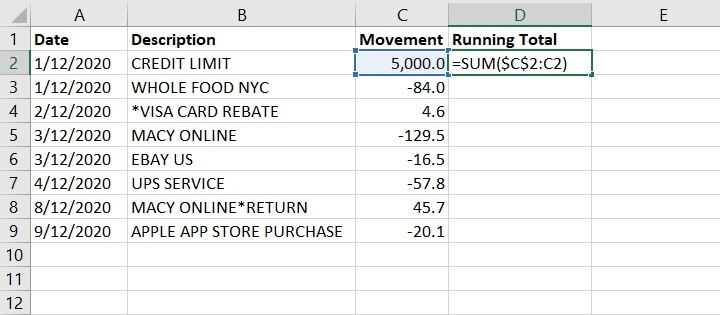Copy the formula and drag it down to apply it to the rest of the cells.

As you can see below, the running total in cell D5 calculates the summation of the values from C2 to C5.

The summation will always begin from cell C2 as it has been locked with the \$ sign (absolute reference), and includes any other values between C2 and C5 (a relative reference).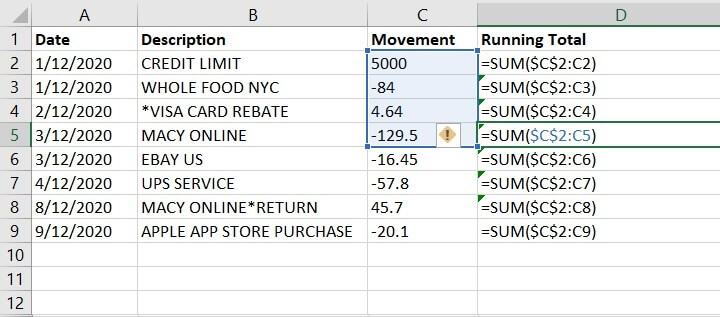Same as the SUM Function, inserting and removing a row will create errors in the formulas and will require manual adjustments.

### 3. Create running total by using Pivot Tables

Pivot Tables are a powerful feature in Excel that allow you to organize, summarize, and analyze tables. It’s commonly used to sort, group, calculate the sum, average or count the values. A Pivot Table can calculate running totals as well.

In a new spreadsheet, create the Pivot Table by using the same set of data.

• Under Rows, add Date and Description
• Under Values, include movement twice. This is so that column C will be showing the daily subtotal and total of the movement. And column D — Sum of Movement 2 will be modified to show running total later.

For easy viewing, the Pivot Table is shown using the outline form below.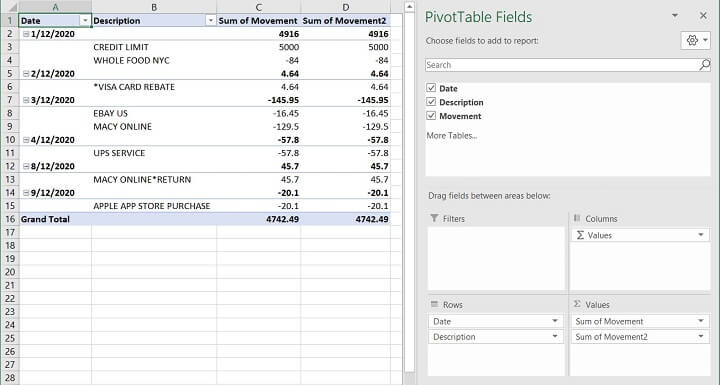In the Pivot Table Fields, right click Sum of Movement 2 to access the menu selection. Click Value Field Settings.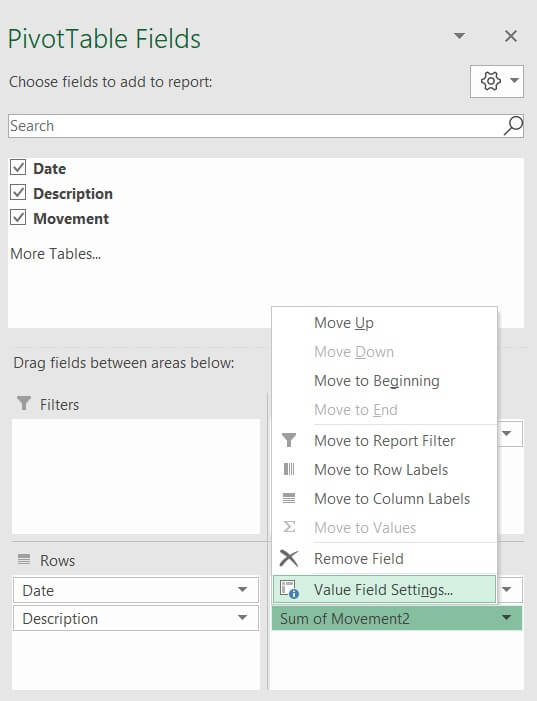The Value Field Settings dialogue box will appear. Plenty of things can be done here.

First, change the name to “Running Total” to differentiate it.

Select Tab — Show Values As, and in the dropdown list, find Running Total In, so the values will be shown as Running Total. In the Base Field, select — Date, as the running total will be performed based on the dates. Click OK.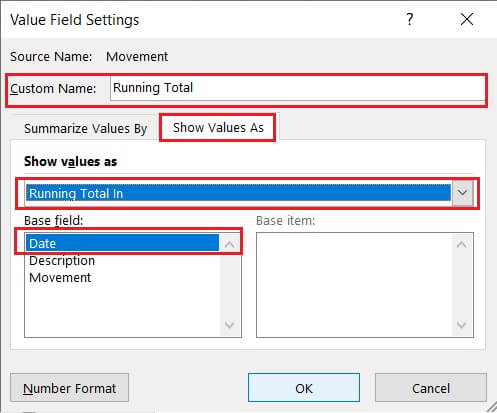Now, the Pivot Table displays the running total of the credit movement by dates. The label of the field is updated as well to Running Total.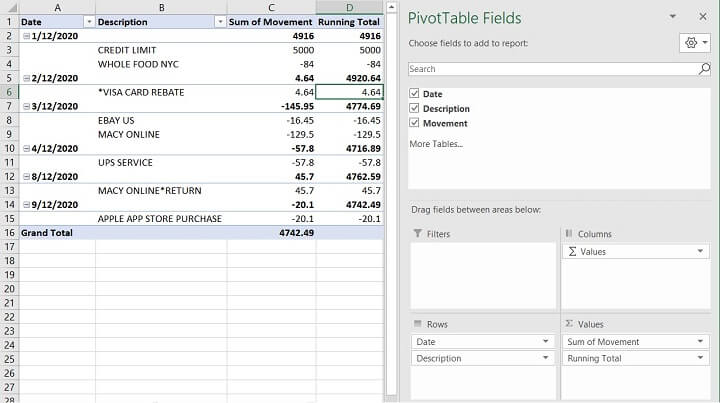In this example, the subtotals are shown at the top of each group. You are free to change it to the bottom of each group if that suits your habits better.

Under the Design Tab, find Subtotals, then click — Show all Subtotals at Bottom of Group.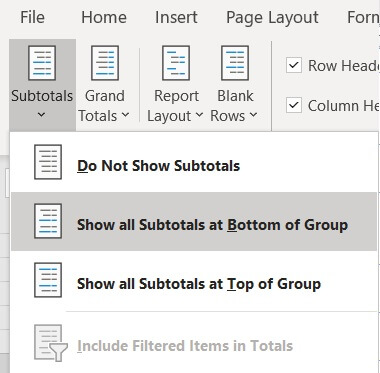Now the Pivot Table displays all the information in an organized manner, detailing the activities by date and the totals of the credit movements and the running total by dates.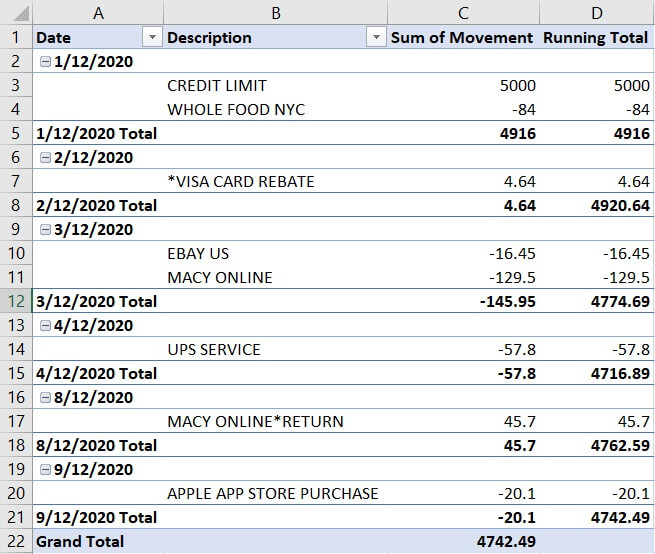If there are any changes to the source data, whether to add or delete a row, simply click refresh, and the table will reflect the changes automatically.

There is no perfect way to create running totals in Excel. All three methods reveal the same results, and each has their own pros and cons. It depends on the complexity of the data set and the calculations on hand.

## Differences on Windows and Mac

The steps required to perform running total on Windows and Mac are the same.

## Summary

Running totals in Excel (also known as cumulative sum) are useful to keep track of progression and changes over time, especially when there is new data coming in or old data being removed from the data set. It’s usually used to monitor sales patterns, bank balance, calorie intake, utility charges and scores of sport games.

There are multiple ways to create running totals in Excel, each with their own pros and cons. It’s important to consider one’s needs and review the data set before choosing the most efficient method to calculate the running total in Excel.

Try the GoSkills Microsoft Excel - Basic and Advanced course today to improve your skills in Excel. Or start with the free Excel in an Hour course to cover some Excel basics.